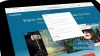Dandy Decimals

Add, Subtract, Multiply, and Divide ·Got Math!

byLisa Arias

ebook

With an OverDrive account, you can save your favorite libraries for at-a-glance information about availability. Find out more about OverDrive accounts.

Subjects

Decimals have never been more fun to learn about than in this rhyming text with step-by-step shortcuts on mastering how to add, subtract, multiply, and divide decimals. Place value, digits, multiplying by powers of ten, models, and practice exercises, all with color-coded answer keys are just a fraction of what will help students learn just how dandy decimals can be! This book will allow students to add, subtract, multiply, and divide decimals to hundredths, using concrete models or drawings and strategies based on place value, properties of operations, and/or the relationship between addition and subtraction.

Publication Details

Publisher:
Rourke Educational Media
Publication Date:
2014
Series:
Got Math!

Format

• Kindle Book
• Adobe PDF eBook 2.4 MB
• Open PDF eBook 2.9 MB
Dandy Decimals
Copy and paste the code into your website.
New here? Learn how to read digital books for freeOR